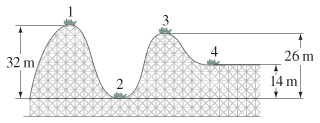# Problem: Suppose the roller-coaster car in the figure passes point 1 with a speed of 3.10 m/s . If the average force of friction is equal to 0.23 of its weight, with what speed will it reach point 2? The distance traveled is 55.0 m .

###### FREE Expert Solution
97% (453 ratings)
###### Problem Details

Suppose the roller-coaster car in the figure passes point 1 with a speed of 3.10 m/s .If the average force of friction is equal to 0.23 of its weight, with what speed will it reach point 2? The distance traveled is 55.0 m .

Frequently Asked Questions

What scientific concept do you need to know in order to solve this problem?

Our tutors have indicated that to solve this problem you will need to apply the Motion Along Curved Paths concept. You can view video lessons to learn Motion Along Curved Paths. Or if you need more Motion Along Curved Paths practice, you can also practice Motion Along Curved Paths practice problems.